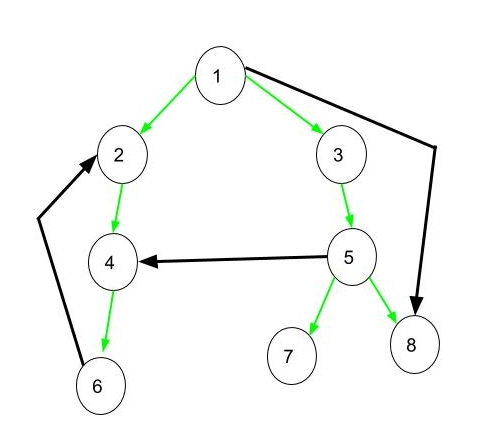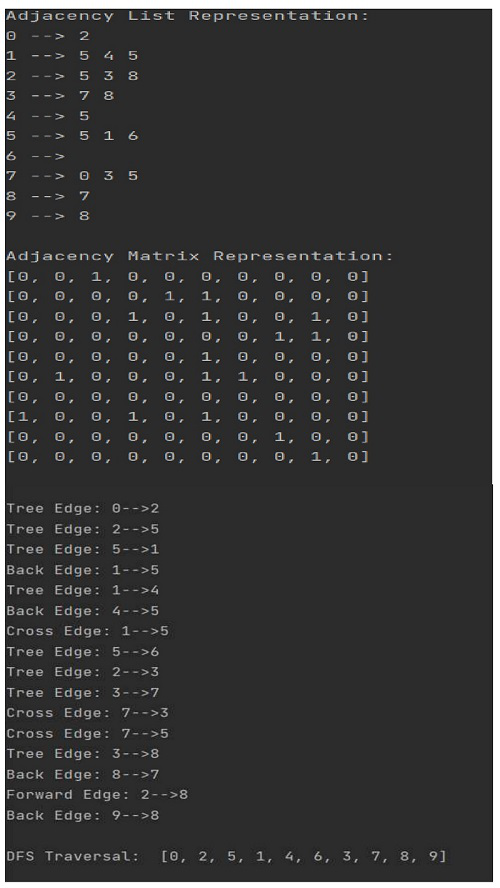# Tree, Back, Edge and Cross Edges in DFS of Graph

• Difficulty Level : Easy
• Last Updated : 12 Dec, 2021

Consider a directed graph given in below, DFS of the below graph is 1 2 4 6 3 5 7 8. In below diagram if DFS is applied on this graph a tree is obtained which is connected using green edges.Tree Edge: It is an edge which is present in the tree obtained after applying DFS on the graph. All the Green edges are tree edges.
Forward Edge: It is an edge (u, v) such that v is descendant but not part of the DFS tree. Edge from 1 to 8 is a forward edge.
Back edge: It is an edge (u, v) such that v is ancestor of node u but not part of DFS tree. Edge from 6 to 2 is a back edge. Presence of back edge indicates a cycle in directed graph
Cross Edge: It is a edge which connects two node such that they do not have any ancestor and a descendant relationship between them. Edge from node 5 to 4 is cross edge.

Time Complexity(DFS)

Since all the nodes and vertices are visited, the average time complexity for DFS on a graph is O(V + E), where V is the number of vertices and E is the number of edges. In case of DFS on a tree, the time complexity is O(V), where V is the number of nodes.

Algorithm(DFS)

Pick any node. If it is unvisited, mark it as visited and recur on all its adjacent nodes.

Repeat until all the nodes are visited, or the node to be searched is found.

#### Example:

Implement DFS using adjacency list take a directed graph of size n=10, and randomly select number of edges in the graph varying from 9 to 45. Identify each edge as forward edge, tree edge, back edge and cross edge.

## Python3

 `# code``import` `random`  `class` `Graph:``    ``# instance variables``    ``def` `__init__(``self``, v):``        ``# v is the number of nodes/vertices``        ``self``.time ``=` `0``        ``self``.traversal_array ``=` `[]``        ``self``.v ``=` `v``        ``# e is the number of edge (randomly chosen between 9 to 45)``        ``self``.e ``=` `random.randint(``9``, ``45``)``        ``# adj. list for graph``        ``self``.graph_list ``=` `[[] ``for` `_ ``in` `range``(v)]``        ``# adj. matrix for graph``        ``self``.graph_matrix ``=` `[[``0` `for` `_ ``in` `range``(v)] ``for` `_ ``in` `range``(v)]` `    ``# function to create random graph``    ``def` `create_random_graph(``self``):``        ``# add edges upto e``        ``for` `i ``in` `range``(``self``.e):``            ``# choose src and dest of each edge randomly``            ``src ``=` `random.randrange(``0``, ``self``.v)``            ``dest ``=` `random.randrange(``0``, ``self``.v)``            ``# re-choose if src and dest are same or src and dest already has an edge``            ``while` `src ``=``=` `dest ``and` `self``.graph_matrix[src][dest] ``=``=` `1``:``                ``src ``=` `random.randrange(``0``, ``self``.v)``                ``dest ``=` `random.randrange(``0``, ``self``.v)``            ``# add the edge to graph``            ``self``.graph_list[src].append(dest)``            ``self``.graph_matrix[src][dest] ``=` `1` `    ``# function to print adj list``    ``def` `print_graph_list(``self``):``        ``print``(``"Adjacency List Representation:"``)``        ``for` `i ``in` `range``(``self``.v):``            ``print``(i, ``"-->"``, ``*``self``.graph_list[i])``        ``print``()` `    ``# function to print adj matrix``    ``def` `print_graph_matrix(``self``):``        ``print``(``"Adjacency Matrix Representation:"``)``        ``for` `i ``in` `self``.graph_matrix:``            ``print``(i)``        ``print``()` `    ``# function the get number of edges``    ``def` `number_of_edges(``self``):``        ``return` `self``.e` `    ``# function for dfs``    ``def` `dfs(``self``):``        ``self``.visited ``=` `[``False``]``*``self``.v``        ``self``.start_time ``=` `[``0``]``*``self``.v``        ``self``.end_time ``=` `[``0``]``*``self``.v` `        ``for` `node ``in` `range``(``self``.v):``            ``if` `not` `self``.visited[node]:``                ``self``.traverse_dfs(node)``        ``print``()``        ``print``(``"DFS Traversal: "``, ``self``.traversal_array)``        ``print``()` `    ``def` `traverse_dfs(``self``, node):``        ``# mark the node visited``        ``self``.visited[node] ``=` `True``        ``# add the node to traversal``        ``self``.traversal_array.append(node)``        ``# get the starting time``        ``self``.start_time[node] ``=` `self``.time``        ``# increment the time by 1``        ``self``.time ``+``=` `1``        ``# traverse through the neighbours``        ``for` `neighbour ``in` `self``.graph_list[node]:``            ``# if a node is not visited``            ``if` `not` `self``.visited[neighbour]:``                ``# marks the edge as tree edge``                ``print``(``'Tree Edge:'``, ``str``(node)``+``'-->'``+``str``(neighbour))``                ``# dfs from that node``                ``self``.traverse_dfs(neighbour)``            ``else``:``                ``# when the parent node is traversed after the neighbour node``                ``if` `self``.start_time[node] > ``self``.start_time[neighbour] ``and` `self``.end_time[node] < ``self``.end_time[neighbour]:``                    ``print``(``'Back Edge:'``, ``str``(node)``+``'-->'``+``str``(neighbour))``                ``# when the neighbour node is a descendant but not a part of tree``                ``elif` `self``.start_time[node] < ``self``.start_time[neighbour] ``and` `self``.end_time[node] > ``self``.end_time[neighbour]:``                    ``print``(``'Forward Edge:'``, ``str``(node)``+``'-->'``+``str``(neighbour))``                ``# when parent and neighbour node do not have any ancestor and a descendant relationship between them``                ``elif` `self``.start_time[node] > ``self``.start_time[neighbour] ``and` `self``.end_time[node] > ``self``.end_time[neighbour]:``                    ``print``(``'Cross Edge:'``, ``str``(node)``+``'-->'``+``str``(neighbour))``            ``self``.end_time[node] ``=` `self``.time``            ``self``.time ``+``=` `1`  `if` `__name__ ``=``=` `"__main__"``:``    ``n ``=` `10``    ``g ``=` `Graph(n)``    ``g.create_random_graph()``    ``g.print_graph_list()``    ``g.print_graph_matrix()``    ``g.dfs()`

### OUTPUT:My Personal Notes arrow_drop_up